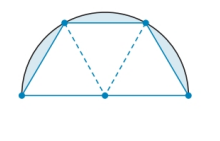Chapter 8.4, Problem 46EElementary Geometry For College St...

7th Edition
Alexander + 2 others
ISBN: 9781337614085

Solutions

Chapter
SectionElementary Geometry For College St...

7th Edition
Alexander + 2 others
ISBN: 9781337614085
Textbook Problem

A tabletop is semicircular when its three congruent drop-leaves are used. By how much has the tables area increased when the drop leaves (shaded area) increased. Give the answer to the nearest whole percent.To determine

To Find:

The percentage of increased area of the tabletop.

Explanation

The tabletop contains three congruent triangles.

The side of the triangle is equal to the radius of the circle.

Therefore, s=r.

The area of the equilateral triangle is A=3s24=3r24.

Therefore, the area of the three triangles is A=33r24.

The area of the semicircle is A=12πr2.

To find the area of the drop-leaves of the tabletop, subtract the area of three triangles from the area of the semicircle

Still sussing out bartleby?

Check out a sample textbook solution.

See a sample solution

The Solution to Your Study Problems

Bartleby provides explanations to thousands of textbook problems written by our experts, many with advanced degrees!

Get Started

Let f(x) = cx + ln(cos x). For what value of c is f(/4) = 6?

Single Variable Calculus: Early Transcendentals, Volume I

Evaluate the integral. 03/2sinxdx

Single Variable Calculus: Early Transcendentals

Sometimes, Always, or Never: fxy = fyx.

Study Guide for Stewart's Multivariable Calculus, 8th

A definite integral for the area of the region bounded by y = 2 − x2 and y = x2 is:

Study Guide for Stewart's Single Variable Calculus: Early Transcendentals, 8th